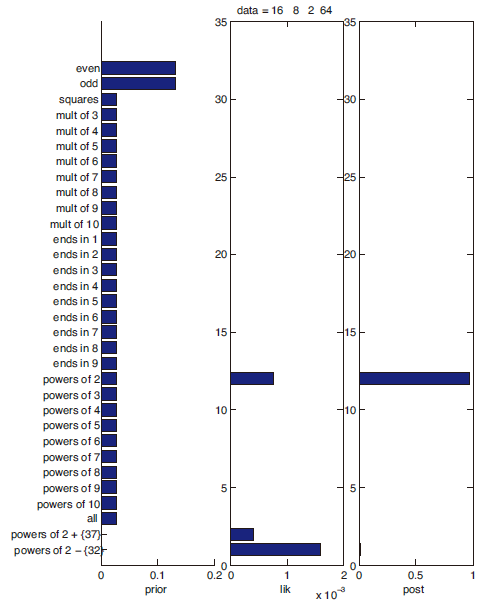Jarvis' Blog 总有美丽的风景让人流连

# 机器学习(二): 离散数据的生成式模型

2018-09-27
Jarvis
Post## 1. 贝叶斯概念学习

### 1.1 似然

• 概率: 指的是概率空间中某个事件发生的概率, 是关于联合样本 $\mathbf{x}$ 的函数
• 似然: 指的是联合样本 $\mathbf{x}$ 关于未知参数 $\theta$ 的似然, 是关于参数 $\theta$ 的函数

$p(\mathcal{D}\lvert h)=\left[\frac{1}{size(h)}\right]^{size(\mathcal{D})}.$

### 1.2 先验和后验

$p(h\lvert\mathcal{D})=\frac{p(\mathcal{D}\lvert h)p(h)}{\sum_{h'\in\mathcal{H}}p(\mathcal{D}, h')}$• “奇数”和”偶数”这两个猜想的先验概率很高, “2 的幂加上 {37}”和”2 的幂减去 {32}”这两个猜想先验概率很低
• 当出现观测值 $\mathcal{D}$ 时, 绝大部分猜想由于与观测值相悖(如: “奇数”)而似然为 0; 而有一些猜想的似然很高(如: “2 的幂次”, “2 的幂次减去 {32}”等), 有一些猜想的似然很低(如: “偶数” 接近 0) .
• 后验概率为第三列, 可以发现两个似然很高的特殊情况”2 的幂次加上 {37}”和”2 的幂次减去 {32}”由于先验很低, 所以最终的后验概率也极低. 而”2 的幂次”这个猜想获得了最高的后验概率.

### 1.3 MLE 和 MAP

• MLE 是寻找一个 $h$ 使得数据集 $\mathcal{D}$ 出现的概率最大
• MAP 是我们(主观的)给出事件的一个先验概率后, 寻找一个 $h$ 使得数据集 $\mathcal{D}$ 出现的概率最大

### 1.4 后验预测分布

• 法一(基于规则的推理 rule-based reasoning): 最直接的想法就是使用数据集 $\mathcal{D}$ 估计该数据集分布的最优参数 $\theta$ , 然后自然根据参数就能得到新数据点 $\tilde{x}$ 的预测值.

$p(\tilde{x}=1\lvert\mathcal{D})= p(\tilde{x}\lvert\theta_{MLE})$

• 法二(基于相似度的推理 similarity-based reasoning): 不把 $\theta$ 固定为一个值, 而是使用概率分布, 即 $\theta$ 以不同的概率取不同的值. 离散形式如下式
$p(\tilde{x}=1\lvert\mathcal{D})=\sum_{\theta}p(x=1\lvert\tilde{x})p(\theta\lvert\mathcal{D}),$

$p(\tilde{x}=1\lvert\mathcal{D})=\int_{\Theta}p(x=1\lvert\theta)p(\theta\lvert\mathcal{D})\,d\theta.$

• 特点: 可以发现当已观测到的数据集 $\mathcal{D}$ 非常小时, 使用插入近似的后验预测分布会变得很窄(即过拟合了); 增大数据集 $\mathcal{D}$ 时, 后验预测分布会逐渐变宽(增加数据减轻过拟合). 使用贝叶斯模型平均方法则后验预测分布会从宽变窄. 而当数据量不断增大, 两种方法最终会收敛到相同的结果.

• 奇数
• 偶数
• 平方数
• 3 的倍数, 4的倍数, …, 10 的倍数
• 以 1 结尾, 以 2 结尾, …, 以 9 结尾
• 2 的幂次, 3 的幂次, …, 10 的幂次
• 所有数据
• 2 的幂次去掉 37
• 2 的幂次 添上 32

[注:] 下次画几张图

## 2. 补充

#### 似然/先验/后验举例

• 3.3 The beta-binomial model
• 3.4 The Dirichlet-multinomial model

Content